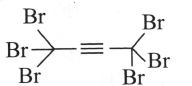Alkynes
Question

# Give the reactivity in the decreasing order of the following alkynes towards nucleophilic addition reaction with ${\mathrm{MeO}}^{\mathrm{\Theta }}/\mathrm{MeOH}$.(I)(II) $\mathrm{Me}-\equiv \mathrm{Me}$(III) $\mathrm{Me}-\equiv -\mathrm{H}$(IV) $\mathrm{H}-\equiv -\mathrm{H}$

Moderate
Solution

## Nucleophilic addition (NA) reaction is favoured by EWG, or easy leaving group or the acidic alkyne in this case.

Get Instant Solutions